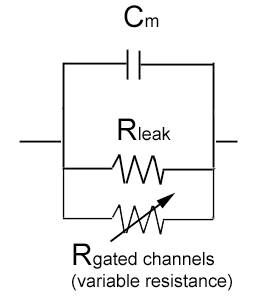# The Neuron Equivalent CircuitThe electrical properties of neurons can described in terms of electrical circuits. This approach helps us understand how a neuron behaves when current flows into it (for example, when ion channels open), or why unmyelinated neurons conduct more slowly than do heavily myelinated neurons.

### The Neuron as RC Circuit

Current can flow across the neuronal membrane through a couple of paths.

Resistors: When there are open ion channels, current can flow through these channels. Each ion channel is a small resistor – and the more open channels in the membrane the more resistors in parallel (so an over all smaller resistance).

The amount of current that flows through these resistors is given by Ohm’s Law:

i = V/R = gV

V is the driving voltage on the ion, which is defined as the difference between membrane potential (Vm) and the reversal potential of the ion (Eion), given by the Nernst Equation. The driving voltage on a given ion species (Na+, or K+ for example) is therefore:

V = Vm – Eion

Conductance (g) is the inverse of the resistance. Conductance increases (resistance decreases) whenever ion channels open, whether due to ligand-binding, voltage changes, or other gating mechanisms.

Capacitor: In electrical circuits, capacitors are structures that are conductor plates separated by a non-conductive material called a dielectric. A voltage across the plates of the conduction plates charges up one plate, pushing charge off the nearby plate. But the plates can only hold so much charge, so current only flows for a short while.

In the cell, the conducting plates are the intracellular and extracellular solutions, separated by the non-conducting membrane. Just like the electrical element, applying a voltage step across the membrane induces a brief current:

i = C dV/dt (current is proportional to the capacitance C and the rate at which voltage changes with time)

The larger the cell the more conducting surface there is apposed to the non-conducting membrane, so the larger the capacitance. A given area of cell membrane has a fixed capacitance (the unit capacitance of cell membrane is approximately 1 microFarad per square cm), so the more membrane the greater the capacitance.

The RC-circuit: The cell membrane can be modeled by a resistor and capacitor in parallel. In the figure, there are two resistors drawn, one is the constitutively open potassium channels that make up the leak current and are the dominant determinant of the resting membrane potential (Rleak). The other is a “variable” resistor that represents other ion channels that can be opened when their gate is activated (for example, by voltage-gating or ligand-gating).

### Time ConstantWhenever there is a step change in voltage across a membrane (or a step current injected across the membrane) it always takes some time to reach a steady state response. Because the cell never completely reaches steady state (it approaches it asymptotically) we instead measure the time it takes to reach most of the way (about 63% of the way) to its final value: the time it takes to get 63% is called the time constant, tau.

For any circuit composed of a resistor and a capacitor (an RC circuit) the time constant is equal to:

tau = RC

So a neuron’s time constant increases with resistance (and decreases with the number of open ion channels) and increases with its surface area since capacitance is proportional to the surface area of the membrane (so larger cells take longer to reach steady state than do smaller cells).

### Length Constant

Changes in membrane potential typically start at a single location. Experimentally this is usually the site in which current is injected through a stimulating electrode. In vivo, depolarization usually begins at the post-synaptic terminal, where neurotransmitter gates ion channels and Na+ enters the cell.

Membrane potential changes propagate along the cell, but not forever. If there are many ion channels open along the membrane, the membrane depolarization will cause current to flow across the membrane which, in the absence of a regenerative action potential, will cause the membrane potential to fall back to resting membrane potential.

The distance that a depolarization can “travel” from the site of initiation is determined by the cell’s length constant. The length constant is derived from “cable theory” which was designed to show explain how an electrical signal degrades along a cable (in this case the cable is the dendrite or axon of the neuron).

The length constant increases with membrane resistance (Resistance Membrane) – that is the fewer paths for current to flow across the membrane, the less the depolarization dissipates. It also depends on the resistance to ion flow along the long axis of the axis (Resistance Axial) – the lower the axial resistance the further the depolarization can travel along the neurite without dissipating. So, the length constant is proportional to:

L = k sqrt( Rm / Ra) (length constant is proportional to the square root of the membrane resistance divided by the axial resistance)